## Compare Predictive Performance After Creating Models Using Econometric Modeler

This example shows how to choose lags for an ARIMA model by comparing the AIC values of estimated models using the Econometric Modeler app. The example also shows how to compare the predictive performance of several models that have the best in-sample fits at the command line. The data set `Data_Airline.mat` contains monthly counts of airline passengers.

### Import Data into Econometric Modeler

At the command line, load the `Data_Airline.mat` data set.

`load Data_Airline`

To compare predictive performance later, reserve the last two years of data as a holdout sample.

```fHorizon = 24; HoldoutTimeTable = DataTimeTable((end - fHorizon + 1):end,:); DataTimeTable((end - fHorizon + 1):end,:) = [];```

At the command line, open the Econometric Modeler app.

`econometricModeler`

Alternatively, open the app from the apps gallery (see Econometric Modeler).

Import `DataTimeTable` into the app:

1. On the Econometric Modeler tab, in the Import section, click the button.

2. In the Import Data dialog box, in the Import? column, select the check box for the `DataTimeTable` variable.

3. Click .

The variable `PSSG` appears in the Time Series pane, its value appears in the Preview pane, and its time series plot appears in the Time Series Plot(PSSG) figure window.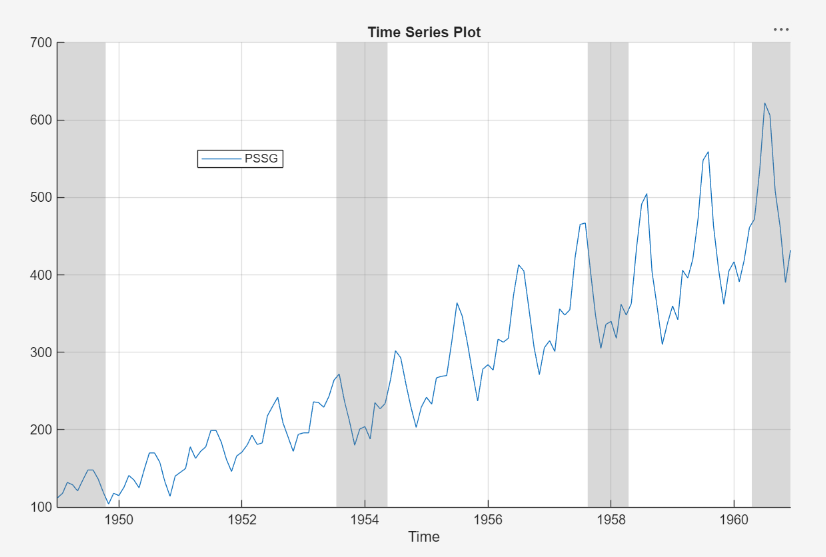The series exhibits a seasonal trend, serial correlation, and possible exponential growth. For an interactive analysis of serial correlation, see Detect Serial Correlation Using Econometric Modeler App.

### Remove Exponential Trend

Address the exponential trend by applying the log transform to `PSSG`.

1. In the Time Series pane, select `PSSG`.

2. On the Econometric Modeler tab, in the Transforms section, click .

The transformed variable `PSSGLog` appears in the Time Series pane, its value appears in the Preview pane, and its time series plot appears in the Time Series Plot(PSSGLog) figure window.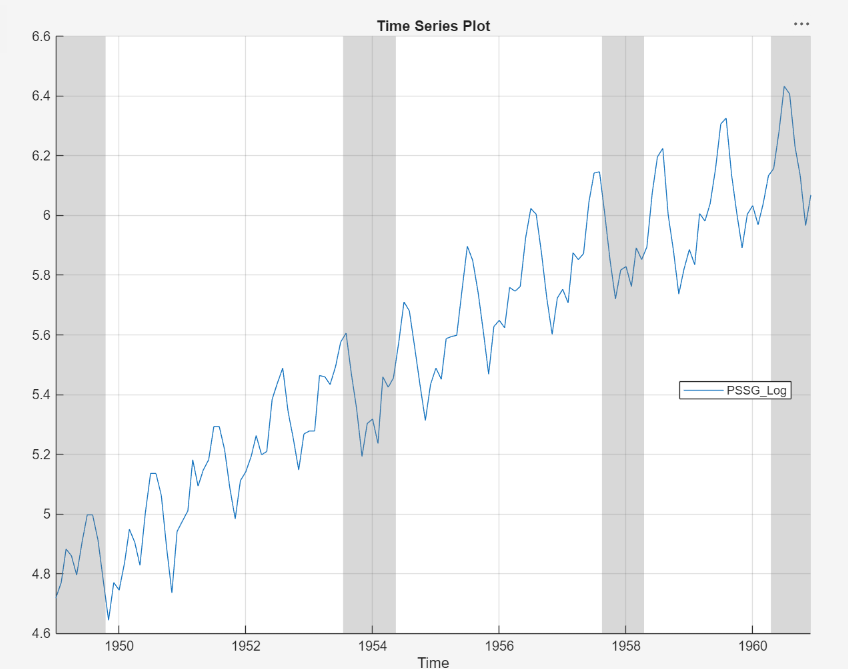The exponential growth appears to be removed from the series.

### Compare In-Sample Model Fits

Box, Jenkins, and Reinsel suggest a SARIMA(0,1,1)×(0,1,1)12 model without a constant for `PSSGLog`  (for more details, see Estimate Multiplicative ARIMA Model Using Econometric Modeler App). However, consider all combinations of monthly SARIMA models that include up to two seasonal and nonseasonal MA lags. Specifically, iterate the following steps for each of the nine models of the form SARIMA(0,1,q)×(0,1,q12)12, where q ∈ {`0`,`1`,`2`} and q12 ∈ {`0`,`1`,`2`}.

1. For the first iteration:

1. Let q = q12 = 0.

2. With `PSSGLog` selected in the Time Series pane, click the Econometric Modeler tab. In the Models section, click the arrow to display the models gallery.

3. In the models gallery, in the ARMA/ARIMA Models section, click SARIMA.

4. In the SARIMA Model Parameters dialog box, on the Lag Order tab:

• Nonseasonal section

1. Set Degrees of Integration to `1`.

2. Set Moving Average Order to `0`.

3. Clear the Include Constant Term check box.

• Seasonal section

1. Set Period to `12` to indicate monthly data.

2. Set Moving Average Order to `0`.

3. Select the Include Seasonal Difference check box.

5. Click .

2. Rename the new model variable.

1. In the Models pane, click the new model variable twice to select its name.

2. Enter `SARIMA01qx01q12`. For example, when `q` = `q12` = 0, rename the variable to `SARIMA010x010`.

3. In the Model Summary(SARIMA01`q`x01`q12`) document, in the Goodness of Fit table, note the AIC value. For example, for the model variable `SARIMA010x010`, the AIC is in this figure.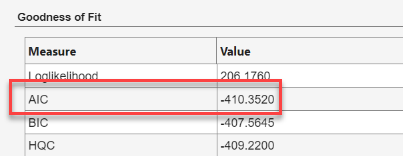4. For the next iteration, choose values of q and q12. For example, q = 0 and q12 = 1 for the second iteration.

5. In the Models pane, right-click `SARIMA01qx01q12`. In the context menu, select Modify to open the SARIMA Model Parameters dialog box with the current settings for the selected model.

6. In the SARIMA Model Parameters dialog box:

1. In the Nonseasonal section, set Moving Average Order to `q`.

2. In the Seasonal section, set Moving Average Order to `q12`.

3. Click .

After you complete the steps, the Models pane contains nine estimated models named `SARIMA010x010` through `SARIMA012x012`.

The resulting AIC values are in this table.

ModelVariable NameAIC
SARIMA(0,1,0)×(0,1,0)12`SARIMA010x010`-410.3520
SARIMA(0,1,0)×(0,1,1)12`SARIMA010x011`-443.0009
SARIMA(0,1,0)×(0,1,2)12`SARIMA010x012`-441.0010
SARIMA(0,1,1)×(0,1,0)12`SARIMA011x010`-422.8680
SARIMA(0,1,1)×(0,1,1)12`SARIMA011x011`-452.0039
SARIMA(0,1,1)×(0,1,2)12`SARIMA011x012`-450.0605
SARIMA(0,1,2)×(0,1,0)12`SARIMA012x010`-420.9760
SARIMA(0,1,2)×(0,1,1)12`SARIMA012x011`-450.0087
SARIMA(0,1,2)×(0,1,2)12`SARIMA012x012`-448.0650

The three models yielding the lowest three AIC values are SARIMA(0,1,1)×(0,1,1)12, SARIMA(0,1,1)×(0,1,2)12, and SARIMA(0,1,2)×(0,1,1)12. These models have the best parsimonious in-sample fit.

### Export Best Models to Workspace

Export the models with the best in-sample fits.

1. On the Econometric Modeler tab, in the Export section, click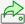.

2. In the Export Variables dialog box, in the Models column, click the Select check box for the `SARIMA011x011`, `SARIMA011x012`, and `SARIMA012x011`. Clear the check box for any other selected models.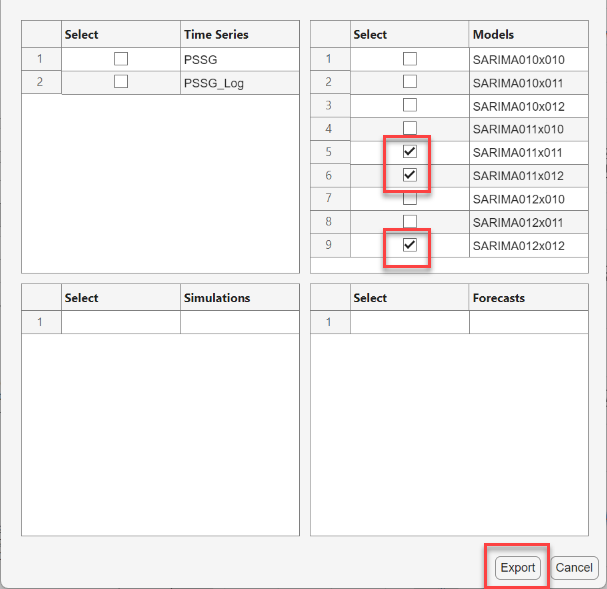3. Click .

The `arima` model objects `SARIMA011x011`, `SARIMA011x012`, and `SARIMA012x011` appear in the MATLAB® Workspace.

### Estimate Forecasts

At the command line, estimate two-year-ahead forecasts for each model.

```f5 = forecast(SARIMA_PSSGLog5,fHorizon); f6 = forecast(SARIMA_PSSGLog6,fHorizon); f8 = forecast(SARIMA_PSSGLog8,fHorizon); ```

`f5`, `f6`, and `f8` are 24-by-1 vectors containing the forecasts.

### Compare Prediction Mean Square Errors

Estimate the prediction mean square error (PMSE) for each of the forecast vectors.

```logPSSGHO = log(HoldoutTimeTable.Variables); pmse5 = mean((logPSSGHO - f5).^2); pmse6 = mean((logPSSGHO - f6).^2); pmse8 = mean((logPSSGHO - f8).^2);```

Identify the model yielding the lowest PMSE.

`[~,bestIdx] = min([pmse5 pmse6 pmse8],[],2)`

The SARIMA(0,1,1)×(0,1,1)12 model performs the best in-sample and out-of-sample.

 Box, George E. P., Gwilym M. Jenkins, and Gregory C. Reinsel. Time Series Analysis: Forecasting and Control. 3rd ed. Englewood Cliffs, NJ: Prentice Hall, 1994.